# mergex()

## CS.mergex()

CS.mergex(xi,…)

CS是对[xi,…]有序的游标的序列，针对其成员输出的对应序列按照表达式xi做有序归并运算。游标中序列的成员必须同构。支持多路游标, 若成员是多路游标，则必须路数相同且同步分段。

 @u 并集运算，CS游标序列的成员按顺序合并到一起组成新的游标，去掉重复的成员。缺省包含所有重复成员。 @i 交集运算，返回CS游标序列中共同的成员组成的游标 @d 差集运算，从游标CS1中去掉游标CS2、…CSn中的成员后形成的新游标 @0 null排最后 @x 从游标CS1、CS2、...CSn中取出不重复的成员组成的新游标

 CS 游标组成的序列 xi 表达式，如果是按照多字段归并，字段间用逗号分隔，例如:x1,x2...

 A B C 1 =demo.cursor("select * from STOCKRECORDS") 返回取数游标 2 for 3 =A1.fetch(500) 游标中取数 4 if B3==null break 5 else 6 =B3.sort(STOCKID) 按STOCKID排序 7 =file("D:\\"+"a"+string(A2)+".txt").export@t(C6) 每次取的数据存到文件中 8 =B1=B1|file("D:\\"+"a"+string(A2)+".txt") 文件对象序列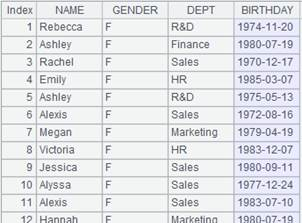9 for B1 10 =A9.cursor@t() 11 =C1=C1|B10 文件游标序列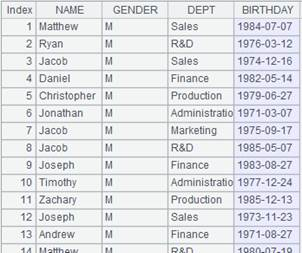12 =C1.mergex(STOCKID) 对序列成员按照成员中的STOCKID有序归并 13 =A12.fetch() 获取合并后的记录 14 =directory@p("D://*.txt") txt文件内容如下：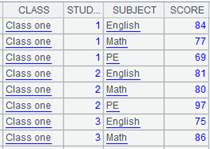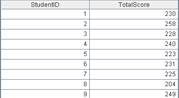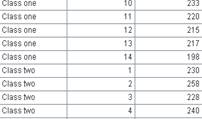15 for A14 16 =file(A15).cursor@t() =B14=B14|B16 17 =B14.mergex@i(STOCKID) =A17.fetch()返回共同成员组成的游标，STOCKID有序 18 =directory@p("D://*.txt") txt内容同上 19 for A18 20 =file(A19).cursor@t() =B18=B18|B20 21 =B18.mergex@u(STOCKID) =A21.fetch()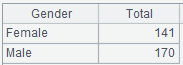返回去掉重复的成员后的新游标，STOCKID有序 22 =directory@p("D://*.txt") txt内容同上 23 for A22 24 =file(A23).cursor@t() =B22=B22|B24 25 =B22.mergex@d(STOCKID) =A25.fetch()从第一个游标中去掉其他游标的成员后形成的新游标，STOCKID有序 26 =directory@p("D://*.txt") 27 for A26 28 =file(A27).cursor@t() =B26=B26|B28 29 =B26.mergex@x(STOCKID) =A29.fetch()取出所有游标中不重复的成员形成的新游标，STOCKID有序

A.merge()

## cs.mergex()

cs.mergex(xi,…)

cs是对[xi,…]有序的集群游标序列，将对应的集群游标按照表达式xi做有序归并运算。组表返回多路游标时，无参数按维归并。

 cs 集群游标组成的序列 xi 表达式，如果是按照多字段归并，字段间用逗号分隔，例如:x1,x2...

 A 1 =file("emp2.ctx":,["127.0.0.1:8282"]) 打开集群文件 2 =A1.open() 打开集群组表 3 =A2.cursor(EID,NAME;EID<8) 返回集群游标4 =A2.cursor(EID,NAME;EID>495) 返回集群游标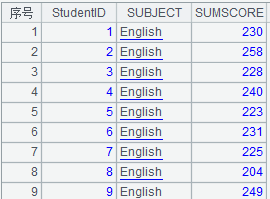5 =[A3,A4].mergex(EID) 将A3和A4按照EID有序合并返回本地单路游标 6 =A5.fetch()A 1 [192.168.0.110:8281,192.168.18.143:8281] 2 =file("emp.ctx":[2,3], A1) 3 =A2.open() 打开集群组表 4 =A3.cursor@m(;;3) 返回集群多路游标 5 =A4.mergex() 组表返回多路游标时，无参数按维归并

## mcs.mergex ()

mcs.mergex(xi,…)

mcs是对[xi,…]有序的多路游标序列，将对应的多路游标按照xi做有序归并运算。其中多路游必须相同路数且同步分段。当mcs是组表多路游标时，自动用维字段归并。

 mcs 多路游标组成的序列 xi 表达式，如果是按照多字段归并，字段间用逗号分隔，例如:x1,x2...

 A 1 =demo.query("select EID,NAME,GENDER,SALARY from EMPLOYEE where EID<6")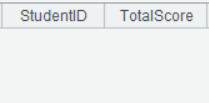EID有序 2 =demo.query("select EID,NAME,GENDER,SALARY from EMPLOYEE where EID<10 and EID>5")EID有序 3 =[A1.cursor(),A2.cursor()].mcursor() 转成多路游标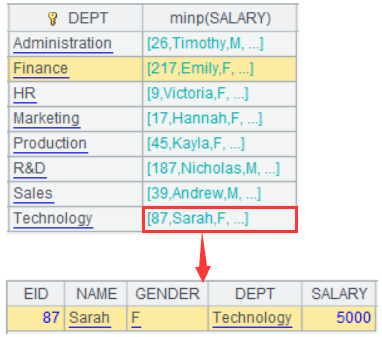4 =[A1.cursor(),A2.cursor()].mcursor() 同A3 5 =[A3,A4].mergex(EID) 将A3和A4按照EID有序合并 6 =A5.fetch()